# 散列表中维护了一个数组，数组的每一个元素被称为一个桶（bucket），当你传入一个key

“a”进行查询时，散列表会先把key传入散列（hash）函数中进行寻址，得到的结果就是数组的下标，然后再通过这个下标访问数组即可得到相关联的值。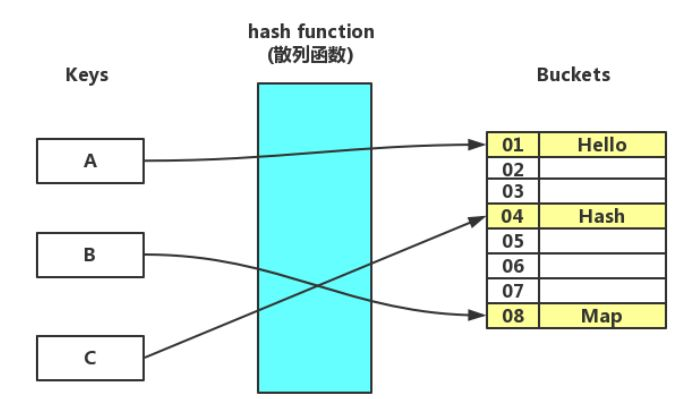hash算法是一种可以从任何数据中提取出其“指纹”的数据摘要算法，它将任意大小的数据（输入）映射到一个固定大小的序列（输出）上，这个序列被称为hash
code、数据摘要或者指纹。比较出名的hash算法有MD5、SHA。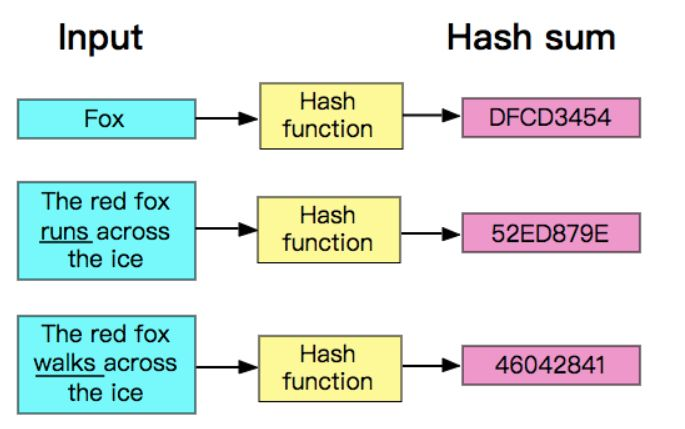hash是具有唯一性且不可逆的，唯一性指的是相同的输入产生的hash
code永远是一样的，而不可逆也比较容易理解，数据摘要算法并不是压缩算法，它只是生成了一个该数据的摘要，没有将数据进行压缩。压缩算法一般都是使用一种更节省空间的编码规则将数据重新编码，解压缩只需要按着编码规则解码就是了，试想一下，一个几百MB甚至几GB的数据生成的hash
code都只是一个拥有固定长度的序列，如果再能逆向解压缩，那么其他压缩算法该情何以堪？

code可能是相同的），这叫做碰撞冲突。总之，理想很丰满，现实很骨感，散列函数只能尽可能地减少冲突，没有办法完全消除冲突。

code，然后再用该hash
code对buckets数组的元素数量取余，得到的结果就是bucket的下标，这种方法简单高效，也可以当做对集群进行负载均衡的路由算法。

private int hash(Key key) {

// & 0x7fffffff

return (key.hashCode() & 0x7fffffff) %
M;

}

code是一个字符串或别的类型，那么你需要将它转换为整数才能使用除留余数法，不过Java在Object对象中提供了hashCode()函数，该函数返回了一个int值，所以任何你想要放入HashMap的自定义的抽象数据类型，都必须实现该函数和equals()函数，这两个函数之间也遵守着一种约定：如果a.equals(b)
== true，那么a与b的hashCode()也必须是相同的。

code（将hash code乘以一个素数并加上当前循环项的字符）：

/** The value is used for character
storage. */

private final char value[];

/** Cache the hash code for the
string */

private int hash; // Default to
0

public int hashCode() {

int h = hash;

if (h == 0 && value.length > 0)
{

char val[] = value;

for (int i = 0; i < value.length;
i++) {

h = 31 * h + val[i];

}

hash = h;

}

return h;

}

HashMap没有采用这么简单的方法，有一个原因是HashMap中的buckets数组的长度永远为一个2的幂，而不是一个素数，如果长度为素数，那么可能会更适合简单暴力的除留余数法（当然除留余数法虽然简单却并不是那么高效的），顺便一提，时代的眼泪Hashtable就使用了除留余数法，它没有强制约束buckets数组的长度。

HashMap在内部实现了一个hash()函数，首先要对hashCode()的返回值进行处理：

static final int hash(Object key)
{

int h;

return (key == null) ? 0 : (h =
key.hashCode()) ^ (h >>> 16);

}

• 1做与运算（table为buckets数组），得到的结果即是数组的下标。

table.length –

table）。

HashMap

## 1就像是一个低位掩码（这个设计也优化了扩容操作的性能），它和hash()做与操作时必然会将高位屏蔽（因为一个HashMap不可能有特别大的buckets数组，至少在不断自动扩容之前是不可能的，所以table.length

1的大部分高位都为0），只保留低位，看似没什么毛病，但这其实暗藏玄机，它会导致总是只有最低的几位是有效的，这样就算你的hashCode()实现得再好也难以避免发生碰撞。这时，hash()函数的价值就体现出来了，它对hash
code的低位添加了随机性并且混合了高位的部分特征，显著减少了碰撞冲突的发生（关于hash()函数的效果如何，可以参考这篇文章An
introduction to optimising a hashing strategy）。

HashMap的散列函数具体流程如下图：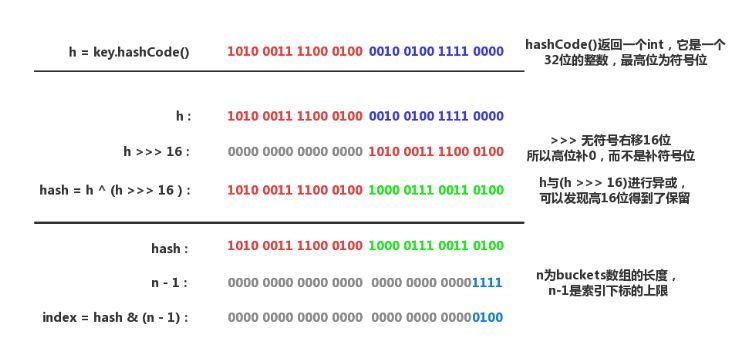chaining，也有人翻译成拉链法）的策略来解决冲突。它的主要思想是每个bucket都应当是一个互相独立的数据结构，当发生冲突时，只需要把数据放入bucket中（因为bucket本身也是一个可以存放数据的数据结构），这样查询一个key所消耗的时间为访问bucket所消耗的时间加上在bucket中查找的时间。

HashMap的buckets数组其实就是一个链表数组，在发生冲突时只需要把Entry（还记得Entry吗？HashMap的Entry实现就是一个简单的链表节点，它包含了key和value以及hash
code）放到链表的尾部，如果未发生冲突（位于该下标的bucket为null），那么就把该Entry做为链表的头部。而且HashMap还使用了Lazy策略，buckets数组只会在第一次调用put()函数时进行初始化，这是一种防止内存浪费的做法，像ArrayList也是Lazy的，它在第一次调用add()时才会初始化内部的数组。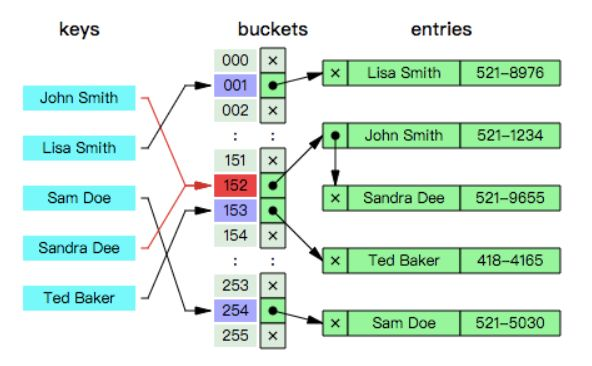8对其实现了优化，链表的节点数量在到达阈值时会转化为红黑树，这样查找所需的时间就只有O(log
n)了，阈值的定义如下：

/**

* The bin count threshold for using a
tree rather than list for a

* bin. Bins are converted to trees
when adding an element to a

* bin with at least this many nodes.
The value must be greater

* than 2 and should be at least 8 to
mesh with assumptions in

* tree removal about conversion back
to plain bins upon

* shrinkage.

*/

static final int TREEIFY_THRESHOLD =
8;

/**

*

*

*/

final void
treeifyBin(Node<K,V>[] tab, int hash) {

int n, index; Node<K,V> e;

// MIN_TREEIFY_CAPACITY =
64，小于该值代表数组中的节点并不是很多

//

if (tab == null || (n = tab.length)
< MIN_TREEIFY_CAPACITY)

resize();

else if ((e = tab[index = (n – 1) &
hash]) != null) {

TreeNode<K,V> hd = null, tl =
null;

//

do {

TreeNode<K,V> p =
replacementTreeNode(e, null);

if (tl == null)

hd = p;

else {

p.prev = tl;

tl.next = p;

}

tl = p;

} while ((e = e.next) != null);

if ((tab[index] = hd) != null)

hd.treeify(tab); //

}

}

// 该函数定义在TreeNode中

final void treeify(Node<K,V>[]
tab) {

TreeNode<K,V> root = null;

for (TreeNode<K,V> x = this,
next; x != null; x = next) {

next =
(TreeNode<K,V>)x.next;

x.left = x.right = null;

if (root == null) { //

x.parent = null;

x.red = false;

root = x;

}

else {

K k = x.key;

int h = x.hash;

Class<?> kc = null;

for (TreeNode<K,V> p = root;;)
{

int dir, ph;

K pk = p.key;

// 确定节点的方向

if ((ph = p.hash) > h)

dir = -1;

else if (ph < h)

dir = 1;

// 如果kc == null

// 并且k没有实现Comparable接口

//

//

else if ((kc == null &&

(kc = comparableClassFor(k)) == null)
||

(dir = compareComparables(kc, k, pk))
== 0)

dir = tieBreakOrder(k, pk);

//

TreeNode<K,V> xp = p;

if ((p = (dir <= 0) ? p.left :
p.right) == null) {

x.parent = xp;

if (dir <= 0)

xp.left = x;

else

xp.right = x;

root = balanceInsertion(root,
x);

break;

}

}

}

}

//

moveRootToFront(tab, root);

}

static int tieBreakOrder(Object a,
Object b) {

int d;

if (a == null || b == null ||

(d = a.getClass().getName().

compareTo(b.getClass().getName())) ==
0)

//
System.identityHashCode()将调用并返回传入对象的默认hashCode()

//

//

d = (System.identityHashCode(a) <=
System.identityHashCode(b) ?

-1 : 1);

return d;

}

addressing），它与分离链接法的思想截然不同。在开放寻址法中，所有Entry都会存储在buckets数组，一个明显的区别是，分离链接法中的每个bucket都是一个链表或其他的数据结构，而开放寻址法中的每个bucket就仅仅只是Entry本身。

hashing，相对的，分离链接法也被称为Open hashing或Closed addressing。

probing），在发生碰撞时，简单地将索引加一，如果到达了数组的尾部就折回到数组的头部，直到找到目标或一个空位。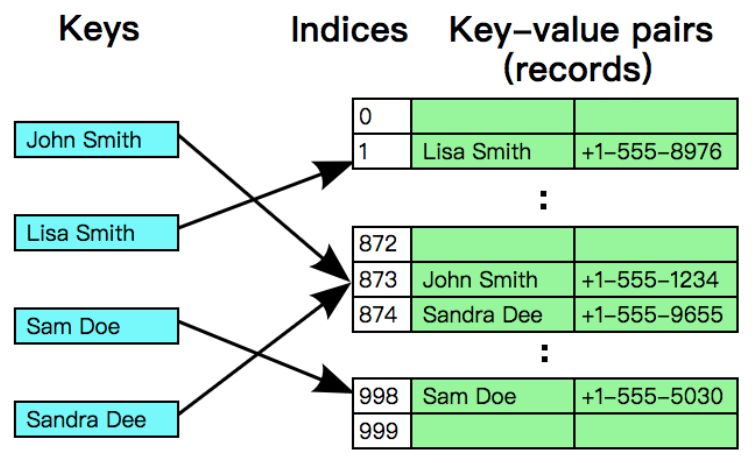private K[] keys; //

private V[] vals; // 存储值的数组

public V get(K key) {

//
m是buckets数组的长度，即keys和vals的长度。

//

for (int i = hash(key); keys[i] !=
null; i = (i + 1) % m) {

if (keys[i].equals(key))

return vals[i];

}

return null;

}

public void put(K key, V value)
{

//
n是Entry的数量，如果n超过了数组长度的一半，就扩容一倍

if (n >= m / 2) resize(2 *
m);

int i;

for (i = hash(key); keys[i] != null;
i = (i + 1) % m) {

if (keys[i].equals(key)) {

vals[i] = value;

return;

}

}

//

keys[i] = key;

vals[i] = value;

n++;

}

public V delete(K key) {

int i = hash(key);

// 先找到目标的索引

while (!key.equals(keys[i])) {

i = (i + 1) % m;

}

V oldValue = vals[i];

// 删除目标key和value

keys[i] = null;

vals[i] = null;

// 指针移动到下一个索引

i = (i + 1) % m;

while (keys[i] != null) {

// 先删除然后重新插入

K keyToRehash = keys[i];

V valToRehash = vals[i];

keys[i] = null;

vals[i] = null;

n–;

put(keyToRehash, valToRehash);

i = (i + 1) % m;

}

n–;

//

if (n > 0 && n <= m / 8) resize(m
/ 2);

return oldValue;

}

HashMap通过负载因子（Load
Factor）乘以buckets数组的长度来计算出临界值，算法：threshold =
load_factor * capacity。比如，HashMap的默认初始容量为16（capacity =
16），默认负载因子为0.75（load_factor = 0.75），那么临界值就为threshold
= 0.75 * 16 = 12，只要Entry的数量大于12，就会触发扩容操作。

/**

*

*
（之前说过buckets数组会推迟到第一次调用put()时进行初始化）

*/

public HashMap(int initialCapacity,
float loadFactor) {

if (initialCapacity < 0)

throw new
IllegalArgumentException(“Illegal initial capacity: ” +

initialCapacity);

if (initialCapacity >
MAXIMUM_CAPACITY)

initialCapacity =
MAXIMUM_CAPACITY;

if (loadFactor <= 0 ||
Float.isNaN(loadFactor))

throw new
IllegalArgumentException(“Illegal load factor: ” +

loadFactor);

this.loadFactor = loadFactor;

//
tableSizeFor()确保initialCapacity必须为一个2的N次方

this.threshold =
tableSizeFor(initialCapacity);

}

buckets数组的大小约束对于整个HashMap都至关重要，为了防止传入一个不是2次幂的整数，必须要有所防范。tableSizeFor()函数会尝试修正一个整数，并转换为离该整数最近的2次幂。

/**

* Returns a power of two size for the
given target capacity.

*/

static final int tableSizeFor(int cap)
{

int n = cap – 1;

n |= n >>> 1;

n |= n >>> 2;

n |= n >>> 4;

n |= n >>> 8;

n |= n >>> 16;

return (n < 0) ? 1 : (n >=
MAXIMUM_CAPACITY) ? MAXIMUM_CAPACITY : n + 1;

}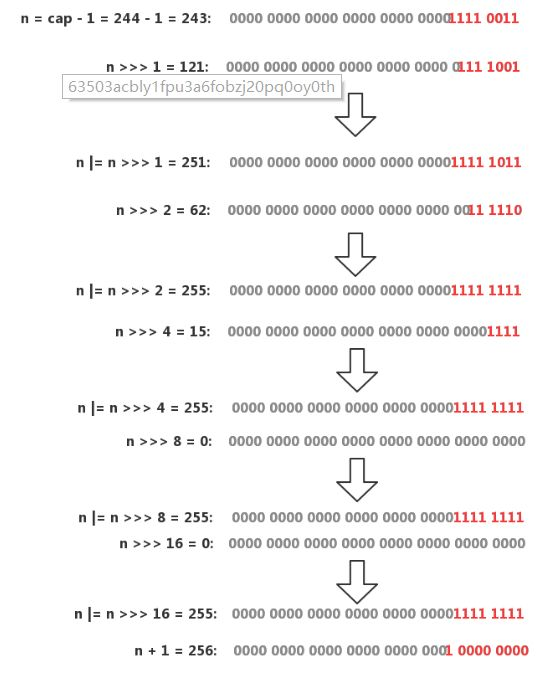hash，这其实是一种优化手段，由于数组的大小永远是一个2次幂，在扩容之后，一个元素的新索引要么是在原位置，要么就是在原位置加上扩容前的容量。这个方法的巧妙之处全在于&运算，之前提到过&运算只会关注n
– 1（n =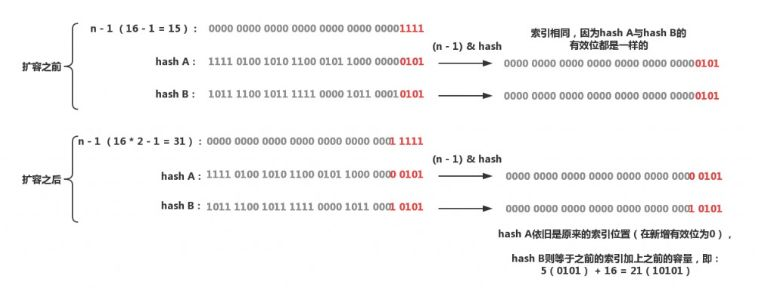final Node<K,V>[] resize()
{

Node<K,V>[] oldTab = table; //
table就是buckets数组

int oldCap = (oldTab == null) ? 0 :
oldTab.length;

int oldThr = threshold;

int newCap, newThr = 0;

//
oldCap大于0，进行扩容，设置阈值与新的容量

if (oldCap > 0) {

//

if (oldCap >= MAXIMUM_CAPACITY)
{

threshold = Integer.MAX_VALUE;

return oldTab;

}

// 没超过最大值，扩容为原来的2倍

// 向左移1位等价于乘2

else if ((newCap = oldCap << 1)
< MAXIMUM_CAPACITY &&

oldCap >=
DEFAULT_INITIAL_CAPACITY)

newThr = oldThr << 1; // double
threshold

}

// oldCap =
0，oldThr大于0，那么就把阈值做为新容量以进行初始化

//

else if (oldThr > 0) // initial
capacity was placed in threshold

newCap = oldThr;

//
oldCap与oldThr都为0，这种情况发生在用户调用了无参构造函数

// 采用默认值进行初始化

else { // zero initial threshold
signifies using defaults

newCap =
DEFAULT_INITIAL_CAPACITY;

newThr = (int)(DEFAULT_LOAD_FACTOR *
DEFAULT_INITIAL_CAPACITY);

}

//

if (newThr == 0) {

float ft = (float)newCap *
loadFactor;

newThr = (newCap < MAXIMUM_CAPACITY
&& ft < (float)MAXIMUM_CAPACITY ?

(int)ft : Integer.MAX_VALUE);

}

threshold = newThr;

style=”font-size: 16px;”>@SuppressWarnings({“rawtypes”,”unchecked”})

Node<K,V>[] newTab =
(Node<K,V>[])new Node[newCap];

table = newTab;

// 如果oldTab !=
null，代表这是扩容操作

//

if (oldTab != null) {

//

for (int j = 0; j < oldCap; ++j)
{

Node<K,V> e;

if ((e = oldTab[j]) != null) {

oldTab[j] = null;

//

// 直接移动到newTab

if (e.next == null)

newTab[e.hash & (newCap – 1)] =
e;

//

//

// 首先会对这个节点进行遍历

// 只要当前节点的hash & oldCap ==
0就链接到lower树

//

//
oldCap就是扩容后新增有效位的掩码

// 比如oldCap=16，二进制10000，n-1 =
1111，扩容后的n-1 = 11111

// 只要hash & oldCap ==
0，就代表hash的新增有效位为0

//

//
lower会被放入newTab[原索引j]，upper树会被放到newTab[原索引j +
oldCap]

//

else if (e instanceof TreeNode)

((TreeNode<K,V>)e).split(this,
newTab, j, oldCap);

else { // preserve order

//

// 只不过split()操作的是TreeNode

//

Node<K,V> loHead = null, loTail =
null;

Node<K,V> hiHead = null, hiTail =
null;

Node<K,V> next;

do {

next = e.next;

if ((e.hash & oldCap) == 0) {

if (loTail == null)

loHead = e;

else

loTail.next = e;

loTail = e;

}

else {

if (hiTail == null)

hiHead = e;

else

hiTail.next = e;

hiTail = e;

}

} while ((e = next) != null);

if (loTail != null) {

loTail.next = null;

newTab[j] = loHead;

}

if (hiTail != null) {

hiTail.next = null;

newTab[j + oldCap] = hiHead;

}

}

}

}

}

return newTab;

}public V put(K key, V value) {

return putVal(hash(key), key, value,
false, true);

}

final V putVal(int hash, K key, V
value, boolean onlyIfAbsent,

boolean evict) {

Node<K,V>[] tab;
Node<K,V> p; int n, i;

// table == null or table.length ==
0

// 第一次调用put()，初始化table

if ((tab = table) == null || (n =
tab.length) == 0)

n = (tab = resize()).length;

// 没有发生碰撞，直接放入到数组

if ((p = tab[i = (n – 1) & hash]) ==
null)

tab[i] = newNode(hash, key, value,
null);

else {

Node<K,V> e; K k;

//

if (p.hash == hash &&

((k = p.key) == key || (key != null &&
key.equals(k))))

e = p;

// 节点为红黑树

else if (p instanceof TreeNode)

e =
((TreeNode<K,V>)p).putTreeVal(this, tab, hash, key,
value);

// 节点为链表

else {

for (int binCount = 0; ; ++binCount)
{

//

if ((e = p.next) == null) {

p.next = newNode(hash, key, value,
null);

if (binCount >= TREEIFY_THRESHOLD –
1) // -1 for 1st

// 链表过长，转换为红黑树

treeifyBin(tab, hash);

break;

}

// 找到目标节点，退出循环

if (e.hash == hash &&

((k = e.key) == key || (key != null &&
key.equals(k))))

break;

p = e;

}

}

// 节点已存在，替换value

if (e != null) { // existing mapping
for key

V oldValue = e.value;

if (!onlyIfAbsent || oldValue ==
null)

e.value = value;

//
afterNodeXXXXX是提供给LinkedHashMap重写的函数

// 在HashMap中没有意义

afterNodeAccess(e);

return oldValue;

}

}

++modCount;

// 超过临界值，进行扩容

if (++size > threshold)

resize();

afterNodeInsertion(evict);

return null;

}

【关于投稿】